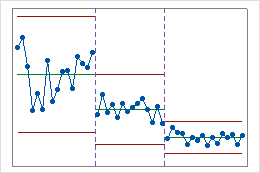# All statistics and graphs for Generalized Variance Chart

Find definitions and interpretation guidance for every statistic and graph that is provided with the Generalized Variance chart.

## Plotted points

The plotted points on a Generalized Variance chart are the generalized variance statistics for each subgroup.

### Interpretation

If the process is in control, the points fall below the upper control limit, and the process exhibits only common-cause variation. Investigate points that fall above the upper control limit. One disadvantage to multivariate charts is that the scale is unrelated to the scale of any of the variables, and out-of-control signals do not reveal which variable (or combination of variables) caused the signal.

## Center line

The center line is the determinant of the sample covariance matrix.

### Interpretation

Use the center line to observe how the process performs compared to the average. If the process is in control, then the points vary randomly around the center line.

## Control limits

Control limits are the horizontal lines that are above and below the center line. The control limits indicate whether a process is out of control, and they are based on the observed variation within subgroups and on the expected variation in the plotted points.

## Covariance matrices

A covariance matrix is a square matrix that contains the variances and covariances for several variables. The diagonal elements of the matrix contain the variances of the variables, and the off-diagonal elements contain the covariances between all possible pairs of variables.

The variance-covariance matrix is symmetric because the covariance between X and Y is the same as the covariance between Y and X. Therefore, the covariance for each pair of variables appears twice in the matrix: the covariance between the ith and jth variables is displayed at positions (i, j) and (j, i).

After you store the covariance matrices, choose Data > Display Data to view the covariance matrices.

### Interpretation

 X Y Z X 2.0 −0.86 −0.15 Y −0.86 3.4 0.48 Z −0.15 0.48 0.82

The variances are displayed in bold along the diagonal. The variance of X, Y, and Z are 2.0, 3.4, and 0.82 respectively. The covariance between X and Y is −0.86, the covariance between X and Z is −0.15, and the covariance between Y and Z is 0.48.

## Stages

Use stages to create a historical control chart that shows how a process changes over specific periods of time. By default, Minitab recalculates the center line and control limits for each stage. For more information, go to Add stages to show how a process changed.

### Interpretation

This historical control chart shows three stages of a process, which represent before, during, and after the implementation of a new procedure.By using this site you agree to the use of cookies for analytics and personalized content.  Read our policy